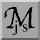jsMath (Test)

If your browser supports JavaScript, be sure it is enabled.

# jsMath Test Page

If you see typeset mathematics below, then jsMath is working. If you see TeX code instead, jsMath is not working for you.

\left(\, \sum_{k=1}^n a_k b_k \right)^2 \le \left(\, \sum_{k=1}^n a_k^2 \right) \left(\, \sum_{k=1}^n b_k^2 \right)

\warning{       jsMath is not working!       }

Once you have jsMath working properly, view the image mode test page to make sure that the image fallback mode is working as well.jsMath web pages Created: 14 Feb 2007 Last modified: Feb 12, 2009 6:14:47 PM Comments to: dpvc@union.edu